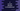# C program to convert decimal to hexadecimal value## C program to convert decimal to hexadecimal value:

In this post, we will learn how to convert decimal to hexadecimal value with an example. Our program will take one number as input from the user and prints out its hexadecimal representation.

Decimal is a base 10 number system and hexadecimal is a base 16 number system.

Decimal number system consists of ten digits 0, 1, 2, 3, 4, 5, 6, 7, 8, 9 and any number can be represented by these digits. This is the most commonly used number system. Again, hexadecimal number system consists of 16 digits 0 to 9 and A to F. 10 in decimal is represented by A and 15 is represented by F.

### How to convert decimal to hexadecimal:

To convert one decimal number to hexadecimal, we need to keep dividing it by 16 and pick the remainder.

For example, Let’s try to convert 1000 to hexadecimal:

• Divide 1000 by 16, 1000/16 = 62, remainder - 8
• 62/16 = 3, remainder - 14
• 3/16 = 0, remadinder - 3

We get the remainders, 3, 14 and 8. hexadecimal value of 14 is E. So, hexadecimal value of 1000 is 3E8

### Decimal to hexadecimal in C algorithm:

So, we need to keep dividing a number by 16 to convert it from decimal to hexadecimal. But in C, we will have to store the result in an character array because the result can be a number or character.

We need to store the integer value as character in the array and this is only possible if we store the ASCII value. ASCII value of 0 is 48 and A is 65. So, if the remainder is less than 10, we can add it with 48 and store it in the character array and if it is more than 9, we will add 55 to it. For example, if it is 10, we will add 55 and it will become 65, which is ASCII of A.

### C program:

Below is the complete C program:

``````#include <stdio.h>
#include <stdlib.h>
#include <string.h>

void getHexadecimalValue(int intValue, char *hexa)
{
int rem, i = 0;

while (intValue != 0)
{
rem = intValue % 16;
intValue = intValue / 16;

if (rem < 10)
{
hexa[i++] = 48 + rem;
}
else
{
hexa[i++] = 55 + rem;
}
}
hexa[i] = '\0';
}
int main()
{
int givenNum;
char *hexaValue;
hexaValue = malloc(sizeof(char) * 50);

printf("Enter a number : ");
scanf("%d", &givenNum);

int strLength = strlen(hexaValue);

for (int j = strLength - 1; j >= 0; j--)
{
printf("%c", hexaValue[j]);
}
printf("\n");
}``````

### Explanation:

• Here, getHexadecimalValue method is used to convert one integer value to hexadecimal.
• It takes two parameters, intValue is the given integer value and one char* to put the hexadecimal converted result.
• Inside main(), we are calling getHexadecimalValue with a user input number givenNum and a char* hexaValue to store the result. The result is copied to hexaValue but it is in reverse order.
• To print the actual hexadecimal converted value, we are using one for loop to iterate the char* in reverse order and printing the characters one by one.